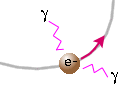Advantages of accelerator designThe advantage of a circular accelerator over a linear accelerator is that the particles in a circular accelerator (synchrotron) go around many times, getting multiple kicks of energy each time around. Therefore, synchrotrons can provide very high-energy particles without having to be of tremendous length. Moreover, the fact that the particles go around many times means that there are many chances for collisions at those places where particle beams are made to cross. On the other hand, linear accelerators are much easier to build than circular accelerators because they don't need the large magnets required to coerce particles into going in a circle. Circular accelerators also need an enormous radii in order to get particles to high enough energies, so they are expensive to build.Another thing that physicists need to consider is that when a charged particle is accelerated, it radiates away energy. At high energies the radiation loss is larger for circular acceleration than for linear acceleration. In addition, the radiation loss is much worse for accelerating light electrons than for heavier protons. Electrons and anti-electrons (positrons) can be brought to high energies only in linear accelerators or in circular ones with large radii. Question: Can an object accelerate while keeping the same speed? [ Answer ]